Texas Go Math Grade 2 Lesson 8.3 Answer Key Model and Record 2-Digit Subtraction

Refer to our Texas Go Math Grade 2 Answer Key Pdf to score good marks in the exams. Test yourself by practicing the problems from Texas Go Math Grade 2 Lesson 8.3 Answer Key Model and Record 2-Digit Subtraction.

Texas Go Math Grade 2 Lesson 8.3 Answer Key Model and Record 2-Digit Subtraction

Explore

Use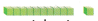to model the problem. Draw quick pictures to show your model.FOR THE TEACHER • Read the following problem. Mr. Kelly brought 47 muffins. His students ate 23 of the muffins. How many muffins were not eaten?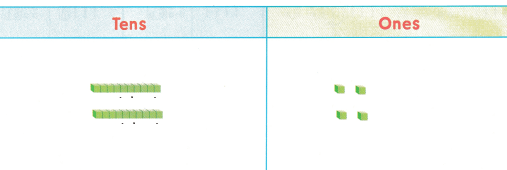Mr. Kelly brought 47 muffins.
His students ate 23 of the muffins.
47 – 23 = 24
24 muffins were not eaten

Math Talk
Mathematical Processes

Yes. 1 line represent the 10
and dot represent the ones

Model and Draw

Subtract.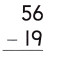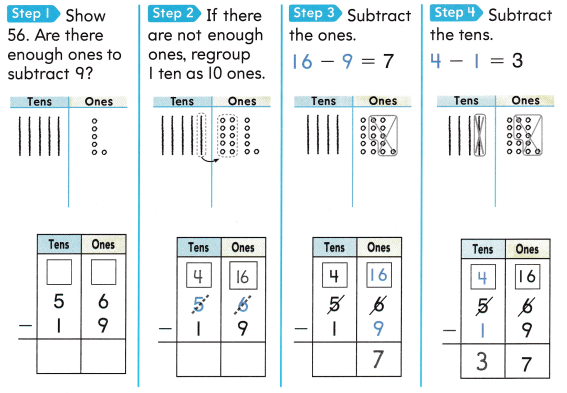Share and Show

Draw a quick picture to solve. Write the difference.

Question 1.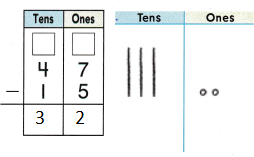Explanation:
When there is no sufficient ones we are regrouping
them tens and subtracting
Again tens are subtracted

Question 2.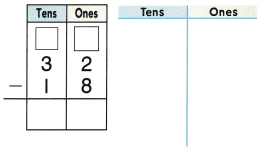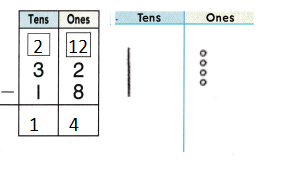Explanation:
When there is no sufficient ones we are regrouping
them tens and subtracting
Again tens are subtracted

Problem Solving

Draw a quick picture to solve. Write the difference.

Question 3.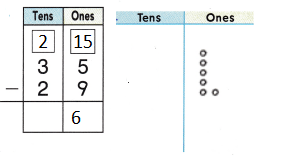Explanation:
When there is no sufficient ones we are regrouping
them tens and subtracting
Again tens are subtracted

Question 4.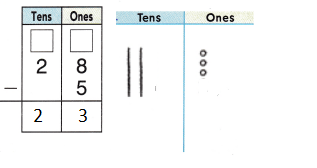Explanation:
When there is no sufficient ones we are regrouping
them tens and subtracting
Again tens are subtracted

Question 5.Explanation:
When there is no sufficient ones we are regrouping
them tens and subtracting
Again tens are subtracted

Question 6.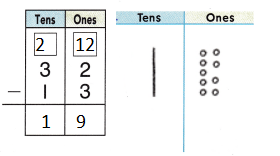Explanation:
When there is no sufficient ones we are regrouping
them tens and subtracting
Again tens are subtracted

Solve. Write or draw to explain.

Question 7.
H.O.T. Claire’s puzzle has 85 pieces. She has used *46 pieces so far. How many puzzle pieces have not been used yet?_________ puzzle pieces
39 puzzle pieces
Explanation:
Claire’s puzzle has 85 pieces.
She has used 46 pieces so far.
85 – 46 = 39
39 puzzle pieces have not been used yet

Question 8.
H.O.T. Multi-Step There are 16 robins in the trees. 24- more fly in. Then 28 robins fly away. How many robins are still in the trees?__________ robins
12 robins
Explanation:
There are 16 robins in the trees.
24- more fly in.
24 + 16 = 40
Then 28 robins fly away.
40 – 28 = 12
12 robins are still in the trees

Question 9.
Rita’s puzzle has 83 pieces. She has already put together 35 pieces. How many pieces does she still have to put together?(A) 58
(B) 48
(C) 51
Explanation:
Rita’s puzzle has 83 pieces.
She has already put together 35 pieces.
83 – 35 = 48
48 pieces that she still have to put together

Question 10.
Analyze A puzzle has l pieces. There are 16 edge pieces. How many pieces are not edge pieces?
(A) 25
(B) 57
(C) 32
Explanation:
A puzzle has 4l pieces.
There are 16 edge pieces.
41 – 16 = 25
25 pieces are not edge pieces

Question 11.
Connect A puzzle has 56 pieces. 38 of them show sky. The rest show grass. How many pieces show grass?
(A) 20
(B) 26
(C) 18
Explanation:
A puzzle has 56 pieces.
38 of them show sky.
The rest show grass.
56 – 38 = 18
18 pieces show grass

Question 12.
TEXAS Test Prep Mr. Sims has a box of 44 erasers. He gives 18 erasers to his students. How many erasers does Mr. Sims have now?(A) 36
(B) 62
(C) 26
Explanation:
Mr. Sims has a box of 44 erasers.
He gives 18 erasers to his students.
44 – 18 = 26
26 erasers that Mr. Sims have now

TAKE HOME ACTIVITY • Write 73-28 on a sheet of paper. Ask your child if he or she would regroup to find the difference.
73 – 38
we cannot subtract 3 – 8
so regrouping is done
73 – 38 = 35

Texas Go Math Grade 2 Lesson 8.3 Homework and Practice Answer Key

Draw a quick picture to solve. Write the difference.

Question 1.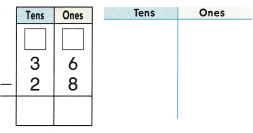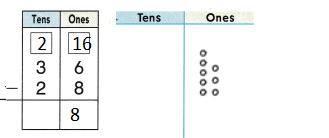Explanation:
When there is no sufficient ones we are regrouping
them tens and subtracting
Again tens are subtracted

Question 2.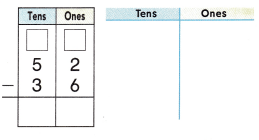Explanation:
When there is no sufficient ones we are regrouping
them tens and subtracting
Again tens are subtracted

Question 3.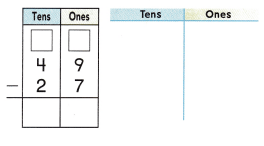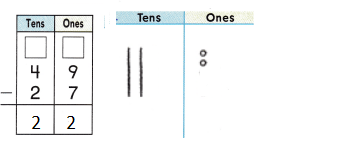Explanation:
When there is no sufficient ones we are regrouping
them tens and subtracting
Again tens are subtracted

Question 4.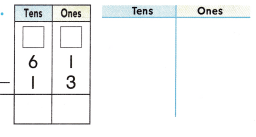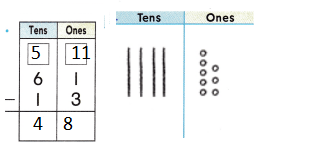Explanation:
When there is no sufficient ones we are regrouping
them tens and subtracting
Again tens are subtracted

Problem Solving

Solve. Write or draw to explain.

Question 5.
Multi-Step There are 15 children at the library story hour. 25 more children join the group. Then 12 children leave. How many children are still at the story hour?
___________ children
38 children
Explanation:
There are 15 children at the library story hour.
25 more children join the group.
15 + 25 = 40
Then 12 children leave.
40 – 12 = 38
38 children are still at the story hour

Lesson Check

Question 6.
Multi-Step Mrs. Norris has a box of 36 buttons. She gives 17 buttons to her daughter. Then she gives 8 buttons to her son. How many buttons does she have now?
(A) 11
(B) 21
(C) 19
Explanation:
Mrs. Norris has a box of 36 buttons.
She gives 17 buttons to her daughter.
Then she gives 8 buttons to her son.
17 + 8 = 25
36 – 25 = 11
11 buttons that she have now

Question 7.
Heather is making a necklace with beads. The necklace will have 75 beads when it is done. The necklace has 37 beads so for. How many beads does Heather still need for the necklace?
(A) 31
(B) 38
(C) 48
Explanation:
Heather is making a necklace with beads.
The necklace will have 75 beads when it is done.
The necklace has 37 beads so for.
75 – 37 = 38
38 beads that Heather still need for the necklace

Question 8.
Paul’s Pizza Palace provides 48 pizza pies for a big party. 19 of the pies have only cheese. The rest of the pies have either vegetables or meat. How many pizza pies have vegetables or meat?
(A) 19
(B) 29
(C) 31
Explanation:
Paul’s Pizza Palace provides 48 pizza pies for a big party.
19 of the pies have only cheese.
The rest of the pies have either vegetables or meat.
48 – 19 = 29
29 pizza pies have vegetables or meat

Question 9.
A crossword puzzle has 4-5 clues. There are 29 across clues. How many are down clues?
(A) 74
(B) 19
(C) 16